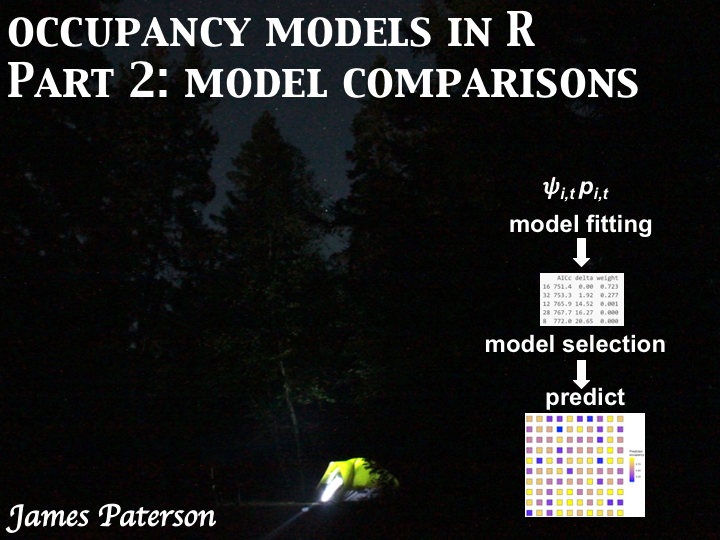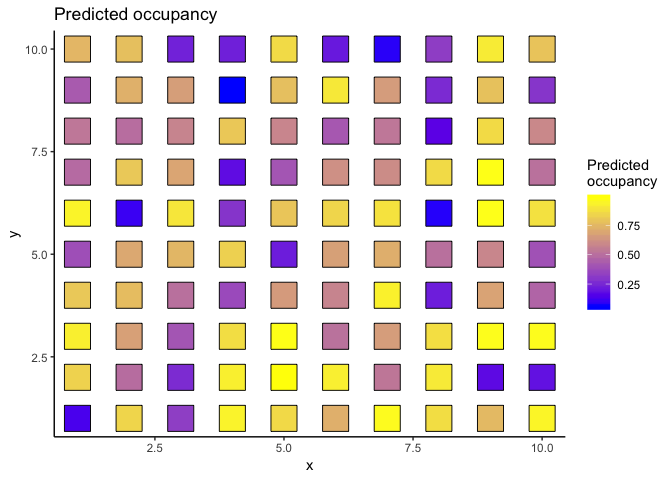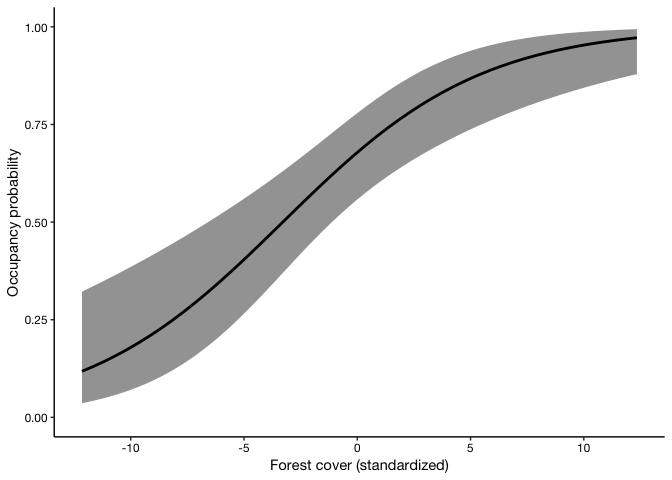In this tutorial, I cover:

1. Fitting and comparing multiple occupancy models with the R package `unmarked`,
2. Model-averaging predictions of occupancy*
3. Model-averaging predicted relationships between occupancy and covariates

*some conditions may apply ;)

The code (and sample data) from this tutorial are available on GitHub.

Let’s get started!

## Occupancy models

As a reminder: occupancy models are useful for estimating species’ distributions and relationships between species occurrence and habitat or landscape variables (among many other things!).

A basic occupancy model estimates two parameters:

• detection probability (\(p_i,_t\), the probability of finding a species at time t if it is present at site i)
• occupancy (\(\Psi_i\), the probability that a species occurs at site i).

In this tutorial, we’re working with static or single-season occupancy models, where the status of a site (occupied or unoccupied) does not change during the study.

## Building multiple single-season occupancy models

In the last tutorial, we covered the basics. But often there are many biologically feasibe models. Let’s use the power of R to systematically build and compare models with different subsets of predictors with the `unmarked` package.

To get started, we need data describing on which surveys that a species was detected. We will use some sample data I simulated. The `unmarked` package uses a special object type to organize detection data and covariates (an `unmarkedFrameOccu` for single season occupancy models).

``````# install.packages("unmarked") # first time only
library(unmarked)
library(dplyr)
library(magrittr)
library(ggplot2)

# Load detection history (100 sites with 10 visits each)
detection_history <- read.csv("detection_history.csv",
# First variable ("X") has row.names but not data
row.names = "X")

# Examine data
head(detection_history)``````
``````##   V1 V2 V3 V4 V5 V6 V7 V8 V9 V10
## 1  0  0  0  0  0  0  0  0  0   0
## 2  1  1  1  0  0  1  1  1  0   1
## 3  1  0  0  0  1  1  0  1  0   0
## 4  1  0  1  1  0  1  0  0  0   1
## 5  0  0  0  0  0  0  0  0  0   0
## 6  0  0  0  0  0  0  0  0  0   0``````
``````# Load covariate data
effort <- read.csv("effort.csv",
# First variable ("X") has row.names but not data
row.names = "X")
observers <- read.csv("observers.csv",
# First variable ("X") has row.names but not data
row.names = "X")
site_cov <- read.csv("site_cov.csv",
# First variable ("X") has row.names but not data
row.names = "X")

# Add a random variable to site_cov (to demonstrate that not all variables should be included in the model)
# We'll assume this variable measures the amount of wetland in some buffer around the site
# Because it is a random number, it shouldn't affect occupancy in our example.
site_cov\$wetland <- rnorm(n = nrow(site_cov), mean = 0, sd = 4)

# Build an unmarkedFramOccu
sample.unmarkedFrame_cov <- unmarkedFrameOccu( # y is a matrix with observed detection history
# 0's and 1's, one row per site, one column per survey
y = as.matrix(detection_history),
# obsCovs = observation covariates in a list,
# each variable has site rows x survey columns
obsCovs = list(effort = effort,
observers = observers),
# siteCovs = dataframe with site rows x column variables
siteCovs = site_cov)

# S4 class for occupancy model data
summary(sample.unmarkedFrame_cov)``````
``````## unmarkedFrame Object
##
## 100 sites
## Maximum number of observations per site: 10
## Mean number of observations per site: 10
## Sites with at least one detection: 62
##
## Tabulation of y observations:
##   0   1
## 703 297
##
## Site-level covariates:
##      sites            forest              agri             wetland
##  Min.   :  1.00   Min.   :-12.1549   Min.   :-11.3766   Min.   :-9.83647
##  1st Qu.: 25.75   1st Qu.: -3.5126   1st Qu.: -2.3297   1st Qu.:-2.11048
##  Median : 50.50   Median : -0.1068   Median :  0.5792   Median :-0.04301
##  Mean   : 50.50   Mean   : -0.1729   Mean   :  0.3977   Mean   :-0.02242
##  3rd Qu.: 75.25   3rd Qu.:  3.5805   3rd Qu.:  3.1949   3rd Qu.: 2.08376
##  Max.   :100.00   Max.   : 12.7956   Max.   : 12.1053   Max.   :10.42488
##
## Observation-level covariates:
##      effort             observers
##  Min.   :-17.284178   Min.   :-15.4163
##  1st Qu.: -3.390206   1st Qu.: -2.7600
##  Median :  0.204287   Median :  0.5063
##  Mean   : -0.007534   Mean   :  0.3759
##  3rd Qu.:  3.200970   3rd Qu.:  3.4080
##  Max.   : 17.784615   Max.   : 15.1235``````

Now that the data are organized, we can build models using `occu()` that includes our various covariates. We’ll start with the general model with all variables.

``````# Fit general model all variables
occu_p_full_psi_full <- occu(formula = ~effort + observers # detection formula first
~forest + agri + wetland, # occupancy formula second,
data = sample.unmarkedFrame_cov)``````

There are a few common approaches to model selection in this type of scenario. With 2 parameter types (detection, occupancy), 2 predictors for detection probability, and 3 predictors for occupancy, there are 32 different possibilities without including interactions between variables. This includes ‘intercept-only’ models (e.g. p~1 or p~dot where detection is the same in every survey). For simplicity, we will test every subset using the `dredge` function.

Note: I’m not giving a blanket recommendation to always do this because it often doesn’t make biological sense. Think very carefully about your general model, and about what subsets you fit if you’re using model selection.

For an interesting read on model selection approaches, check-out Morin et al. 2020 (link in references). Their suggestions are most useful if there are lots (eg hundreds) of potential model subsets.

In our example today, we’re going to assume that the general model fits sufficiently. I covered goodness-of-fit tests in the previous tutorial, but note that the ranking can be switched to QAICc if the variance inflation factor (c-hat) > 1.

``````## Global model call: occu(formula = ~effort + observers ~ forest + agri + wetland,
##     data = sample.unmarkedFrame_cov)
## ---
## Model selection table
##     p(Int) psi(Int) p(eff) p(obs) psi(agr) psi(frs) psi(wtl) df   logLik
## 16 -0.2612   0.8425 0.1397 0.2927  -0.2489   0.2269           6 -369.239
## 32 -0.2617   0.8544 0.1396 0.2927  -0.2563   0.2273 -0.05793  7 -368.936
## 12 -0.2615   0.6210 0.1396 0.2927            0.1744           5 -377.633
## 28 -0.2617   0.6206 0.1396 0.2927            0.1731 -0.03395  6 -377.499
## 8  -0.2618   0.6189 0.1398 0.2928  -0.1627                    5 -380.698
##     AICc delta weight
## 16 751.4  0.00  0.701
## 32 753.1  1.71  0.298
## 12 765.9 14.52  0.000
## 28 767.9 16.52  0.000
## 8  772.0 20.65  0.000
## Models ranked by AICc(x)``````

The model selection table includes the estimates from the model, plus the regular group of model-selectin values (logLik, AICc, delta or difference to most-supported model, and model weight).

We compared all model subsets using AICc, and can conclude that:

1. The most supported model included effort and observers as covariates of p, and agriculture and forest as predictors of occupancy.
2. The general model was the second most-supported of the candidate models (second lowest AICc)
3. No other model had much support (> 10 AICc units away from most-supported model.

This model comparison is useful, but we also want to predict the probability of occupancy at each site, while accounting for model uncertainty using model-averaging.

We can use the `modavgPred` function in the `AICcmodavg` package to predict values using a list of model objects (`occu_model_list` in our example has two models). The function automatically weighs the predictions based on relative support for each model.

``````# With lots of models, it will be slow
library(AICcmodavg)
occu_modavg_psi_predict <- modavgPred(occu_model_list,
# c.hat = 1, # to change variance inflation factor, default = 1)
parm.type = "psi", # psi = occupancy, can also be "det" for detection probability
newdata = sample.unmarkedFrame_cov@siteCovs)[c("mod.avg.pred",
"lower.CL",
"upper.CL")]

## Put predictions, CI, and all site covariates into one data frame
occu_modavg_psi_predict_df <- data.frame(Predicted = occu_modavg_psi_predict\$mod.avg.pred,
lower = occu_modavg_psi_predict\$lower.CL,
upper = occu_modavg_psi_predict\$upper.CL,
site_cov)

# Look at first values
head(occu_modavg_psi_predict_df)``````
``````##   Predicted      lower     upper sites    forest       agri     wetland
## 1 0.1340588 0.03253914 0.4153948     1  0.919871 11.5602018  1.09596909
## 2 0.8456879 0.71221382 0.9240370     2  3.949650  0.2974111 -1.99354691
## 3 0.3261576 0.17478981 0.5240921     3 -5.768212  1.3504457 -4.30822016
## 4 0.9358810 0.77166792 0.9852768     4  4.323499 -4.2356399 10.42488366
## 5 0.8577785 0.73108042 0.9304688     5  1.790066 -2.1722418  0.04597688
## 6 0.7230713 0.56601442 0.8394249     6 -2.590940 -2.8654543  1.02283949``````

Now we have an estimate (with uncertainty) of the probability the target species occurs at each of the study sites. Mapping these predictions can be especially useful. Let’s assume that the sites are in a 10 x 10 grid (100 sites total).

``````occu_modavg_psi_predict_df <- occu_modavg_psi_predict_df %>%
mutate(x = rep(1:10, 10), # Adding pseudo-coordinates as if 100 sites are in 10 x 10 grid
y = rep(1:10, each = 10))

# Note, you will probably plot on map and use polygons, not points
# This is an example just to show how you can easily visualize the predicted occupancy across all sites
ggplot(data = occu_modavg_psi_predict_df, aes(x = x, y = y, fill = Predicted)) +
geom_point(size = 10, pch = 22) +
theme_classic() +
scale_fill_gradient(low = "blue", high = "yellow", name = "Predicted\noccupancy") +
ggtitle("Predicted occupancy")``````What about relationships with covariates? We can use model-averaging to look at how occupancy is predicted to change with our covariates. Below, we’ll predict the relationship between occupancy and forest while accounting for model uncertainty.

``````# First, set-up a new dataframe to predict along a sequence of the covariate.
# Predicting requires all covariates, so let's hold the other covariates constant at their mean value
occu_forest_newdata <- data.frame(forest = seq(min(site_cov\$forest),
max(site_cov\$forest), by = 0.5),
agri = mean(site_cov\$agri), # hold other variables constant
wetland = mean(site_cov\$wetland)) # hold other variables constant

# Model-averaged prediction of occupancy and confidence interval
occu_forest_pred <- modavgPred(occu_model_list,
# c.hat =    # to change variance inflation factor, default = 1)
parm.type = "psi", # psi = occupancy
newdata = occu_forest_newdata)[c("mod.avg.pred",
"lower.CL",
"upper.CL")]

# Put prediction, confidence interval, and covariate values together in a data frame
occu_forest_pred_df <- data.frame(Predicted = occu_forest_pred\$mod.avg.pred,
lower = occu_forest_pred\$lower.CL,
upper = occu_forest_pred\$upper.CL,
occu_forest_newdata)

# Plot the relationship
occu_forest_pred_plot <- ggplot(occu_forest_pred_df, aes(x = forest, y = Predicted)) +
geom_ribbon(aes(ymin = lower, ymax = upper), alpha = 0.5, linetype = "dashed") +
geom_path(size = 1) +
labs(x = "Forest cover (standardized)", y = "Occupancy probability") +
theme_classic() +
coord_cartesian(ylim = c(0,1)) +
theme(text = element_text(family = "HelveticaNeue", colour = "black"),
axis.text = element_text(colour = "black"))
occu_forest_pred_plot``````In our example the predictions from a) model-averaging two models and b) just using the most-supported model are almost identical because:

• the most-supported model had much more support then the second candidate model (weight = 0.75 vs 0.25)
• there were only two models in the candidate model set and the coefficients were similar.

In many cases several candidate models are likely to have very similar support, so model-averaging predictions can be useful to account for uncertainty.

That’s all for now, you should take a break!

We have covered fitting mutiple subsets of single-season occupancy models, comparing models with information theoretic criteria, model-averaging predictions of occupancy at sites, and model-averaging relationships between occupancy and covariates.

Next, I’ll introduce dynamic occupancy models where occupancy status changes through time via colonization and extinction.

## Further reading

1. Don’t already have your data in capture-history format? Check-out my past post on creating capture histories.

2. Introduction to occupancy models in R

3. unmarked Package Vignette https://cran.r-project.org/web/packages/unmarked/vignettes/unmarked.pdf

4. AICcmodavg Package documentation https://cran.r-project.org/web/packages/AICcmodavg/index.html

5. Morin et al. 2020. Is your ad hoc model selection strategy affecting your multimodel inference? Ecosphere 11(1): e02997 https://doi.org/10.1002/ecs2.2997